# 二,ESP8266 GPIO和SPI和定时器和串口gpio.mode(4,gpio.OUTPUT)--输出模式
gpio.write(4,0)--输出低电平gpio.mode(2,gpio.OUTPUT)
gpio.write(2,1)gpio.mode(4,gpio.OUTPUT)
gpio.write(4,1)

function aa()--定义一个函数
print("111111")
end

tmr.alarm(0, 1000, 1, aa)
0代表用的标号为0的定时器

1000代表延时1000ms执行aa

1代表tmr.ALARM_AUTOgpio.mode(4,gpio.OUTPUT)
gpio.write(4,1)

tmr.alarm(1, 1000, 1, function()
print("111111")
end)gpio.mode(4,gpio.OUTPUT)
gpio.write(4,1)

tmr.alarm(1, 2000, 0, function()

uart.on("data", 0,function(Revdata)

uart.write(0,Revdata)

end, 0)

end)

uart.on("data", 0,function(Revdata)

uart.write(0,Revdata)

end, 0)

uart.write(0,Revdata)

现在用串口助手测试一下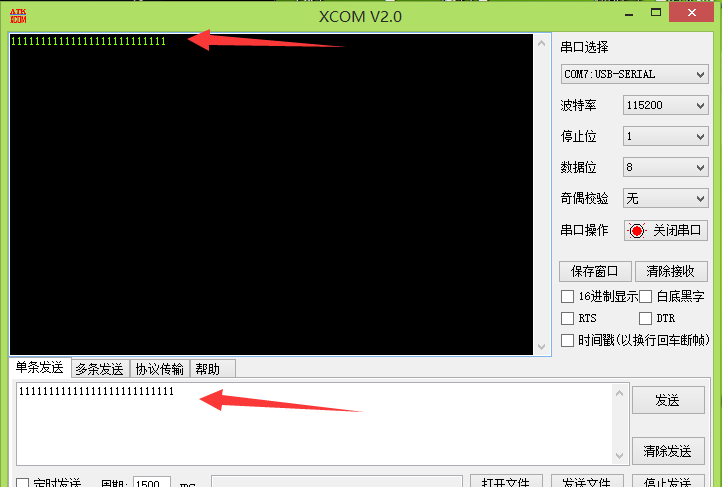现在我假如修改了程序想重新写入芯片

复位芯片后赶紧点击,,当然有两秒时间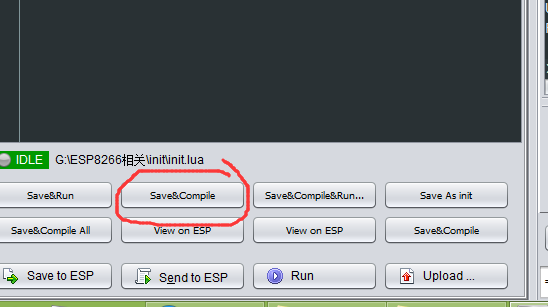如果程序小有时候可以直接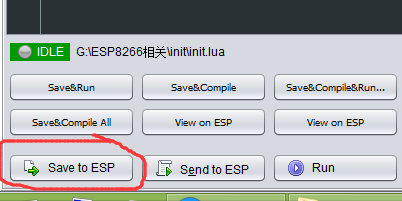如果程序大点,,可以再复位一下模块然后再...当然假设下载的时间不会超过两秒假设程序很大

复位芯片后赶紧点击,,当然有两秒时间然后看这里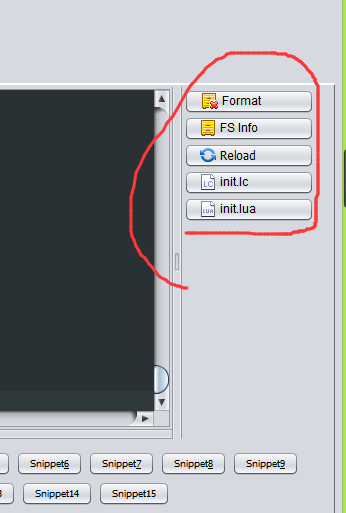只要移除掉init.lua一切都好办

那我先右击准备好点击,,,,然后呢我复位一下模块,然后在两秒之前点击就移除了init.lua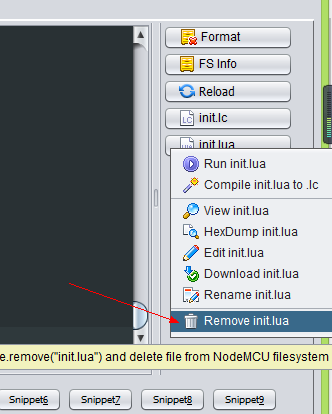还有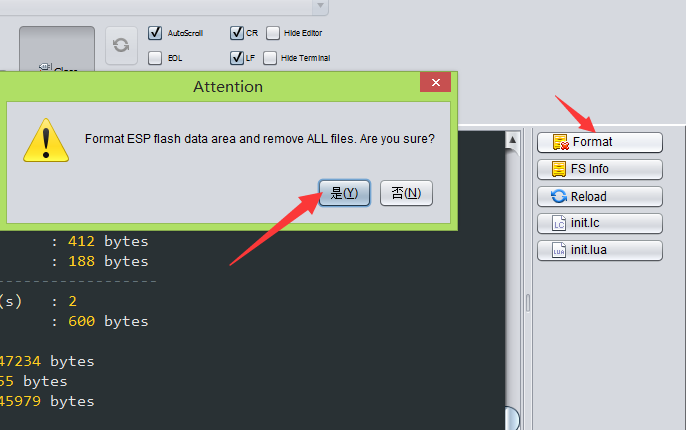也是复位后再点击是,,,移除芯片内部所有文件..........这个随时可以用,,不用像上面似的事先编译

当然这只是在自己配置了串口之后的特殊情况下才会遇到的问题哈

做到这里有些人会想,能不能配置收到什么数据就去干点什么

我们就配置收到H 就控制继电器引脚输出高电平,收到L就控制继电器引脚输出低电平

print("Relay=1")
print("Relay=0")

gpio.mode(4,gpio.OUTPUT)
gpio.mode(2,gpio.OUTPUT)
gpio.write(4,1)

tmr.alarm(1, 2000, 0, function()

uart.on("data", 0,function(Revdata)

if Revdata == "H" then
gpio.write(2,1)
print("Relay=1")
end

if Revdata == "L" then
gpio.write(2,0)
print("Relay=0")
end

uart.write(0,Revdata) -- unregister callback function

end, 0)

end)gpio.mode(4,gpio.OUTPUT)
gpio.mode(2,gpio.OUTPUT)
gpio.write(4,1)

tmr.alarm(1, 2000, 0, function()

uart.on("data", 0,function(Revdata)

if Revdata == "++H" then
gpio.write(2,1)
print("Relay=1")
end

if Revdata == "++L" then
gpio.write(2,0)
print("Relay=0")
end

uart.write(0,Revdata) -- unregister callback function

end, 0)

end)gpio.mode(4,gpio.OUTPUT)
gpio.mode(2,gpio.OUTPUT)
gpio.write(4,1)

tmr.alarm(1, 2000, 0, function()

uart.on("data", 0,function(Revdata)

if Revdata == "++H" then
gpio.write(2,1)
print("Relay=1")
else
print(Revdata)----LOOK  LOOK  LOOK
end

if Revdata == "++L" then
gpio.write(2,0)
print("Relay=0")
end

uart.write(0,Revdata) -- unregister callback function

end, 0)

end)gpio.mode(4,gpio.OUTPUT)
gpio.mode(2,gpio.OUTPUT)
gpio.write(4,1)

tmr.alarm(2, 5, 1, function()

if  ReadCnt ~= 0 then

if  ReadDataCopy == "++H" then
gpio.write(2,1)
end

if  ReadDataCopy == "++L" then
gpio.write(2,0)
end

else
end

end

end)

tmr.alarm(1, 2000, 0, function()

uart.on("data", 0,function(Revdata)

end, 0)

end)https://item.taobao.com/item.htm?spm=686.1000925.0.0.4a155084OQhC4a&id=558508797404